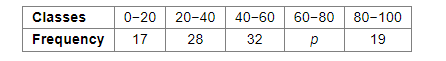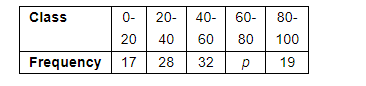# The mean of the following frequency`
Question:

The mean of the following frequency distribution is 50. Find the value of p.Solution:

Given that the mean value of the following distribution is 50.We have to find the value of p.

To find the value of p, we have following procedureThus, we have

$\sum f_{i}=96+p$

$\sum f_{i} x_{i}=4320+70 p$

We know that the formula of mean is given by

mean $=\frac{\sum f_{i} x_{i}}{\sum f_{i}}$

$\Rightarrow \quad 50=\frac{4320+70 p}{96+p}$

$\Rightarrow 4800+50 p=4320+70 p$

$\Rightarrow \quad 20 p=480$

$\Rightarrow \quad p=24$

Hence $p=24$